• Call Now

1800-102-2727•

# Gamma Decay: Definition, Examples, Practice Problems and FAQs

The term “ gamma rays” was coined by Ernst Rutherford, who also conducted the famous gold-foil experiment. He conducted vast experiments and observed that unstable nuclei become stable by following a phenomenon called radioactive decay or radioactivity wherein they emit certain subatomic particles (like alpha, beta). Rutherford observed that alpha particles, when allowed to pass through a metal foil, were stopped after a certain distance of thickness of foil; beta particles were found to have penetrating power a hundred times more than that of alpha particles. He observed a third type of radiation, which had far greater penetrating power, and he called them gamma rays. Today, these “ gamma rays” find wide applications in medical and scientific areas. So what exactly are gamma rays, and when are they emitted? Let’s explore!

## Definition of Gamma Decay

Whenever an excited nucleus emits an alpha or beta particle, the daughter nucleus goes to an excited state. The excited nucleus returns to its ground state and in the process, it emits a gamma ray(y). A gamma ray or gamma photon is an electromagnetic radiation having high energy. If E denotes energy of a gamma ray, and indicates the frequency of the gamma photon, then E=hv , where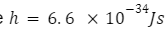indicates Planck’s constant.

Let Enf indicate the final energy level of the nucleus in its excited state and Eni indicate the initial energy level at its ground state. The difference between the two energy levels manifests itself as a photon of frequency f.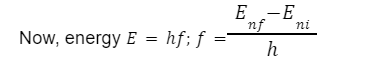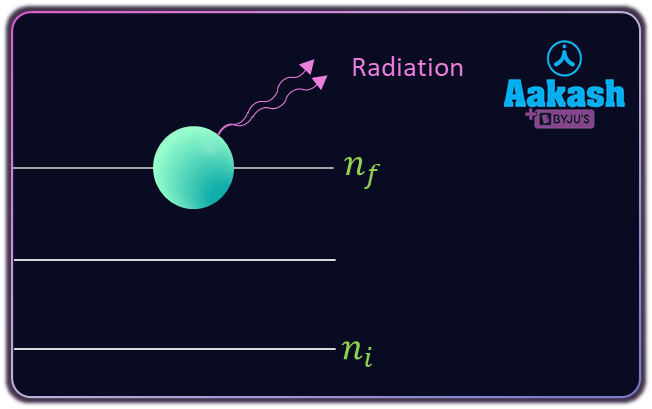Fig showing the initial and final energy levels. Gamma radiation

is emitted in the process.

## Example of Gamma Decay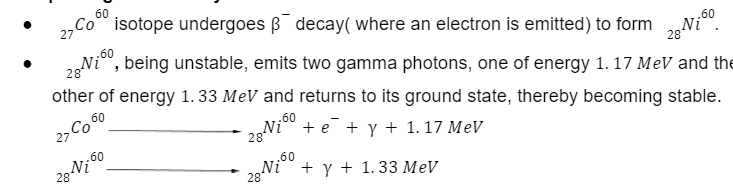• When a nucleus undergoes gamma decay, there is no change in its atomic number or mass number
•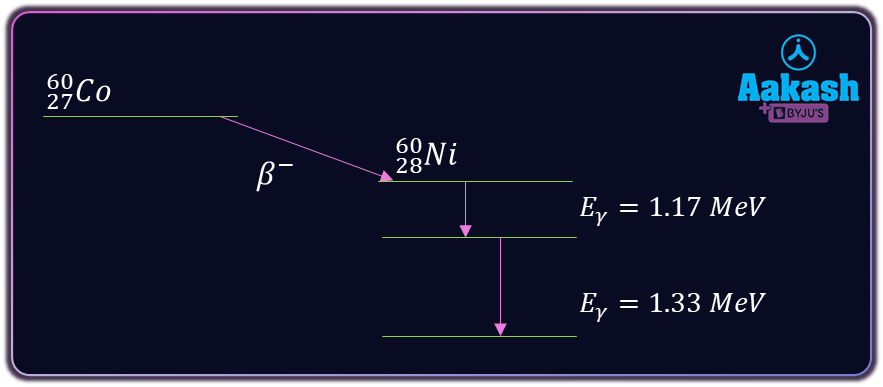## Properties of Gamma Rays

• y - rays do not have charge, so they are not deflected by electric and magnetic fields.
• They propagate with the same speed as that of light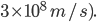.
• They have higher penetrating power than or particles. The order of penetrating power is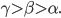.
• y- rays ionize gases. The order of ionizing power is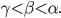• y- rays produce fluorescence.
• They affect photographic plates.
• y- rays undergo diffraction, when they are passed through crystals.

## Practice Problems of Gamma Decay

Question.1 Calculate the minimum frequency of ay - photon required to cause a deuteron atom to disintegrate into a proton and a neutron (md=2.0141 amu, mn=1.0087 amu, mp=1.0078 amu)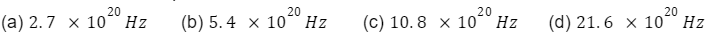Let v indicate the frequency of the photon. Then the energy of the gamma photon emitted, E=hv.

Also, energy released=mass defect c2, where c=3 108 m/s indicates the speed of light.

Mass defect=(1.0087+1.0078)-(2.0141)=2.0165-2.0141=0.0024 amu

Now, 1 amu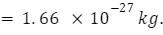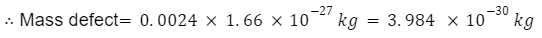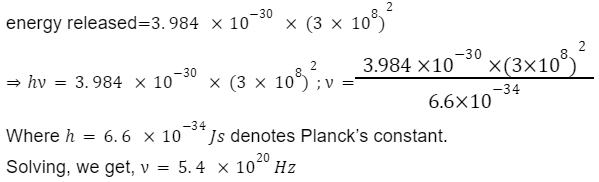Question.2 A free nucleus of mass 24 amu emits a gamma photon while initially it is at rest. The energy of the photon is found to be 7 MeV. The recoil energy of the nucleus, in keV is

(a) 2.2 (b)1.1 (c) 3.1 (d)22

Given, mass of the nucleus, m=24 amu.

Let Ey indicate the energy of the emitted gamma photon and py indicate its momentum.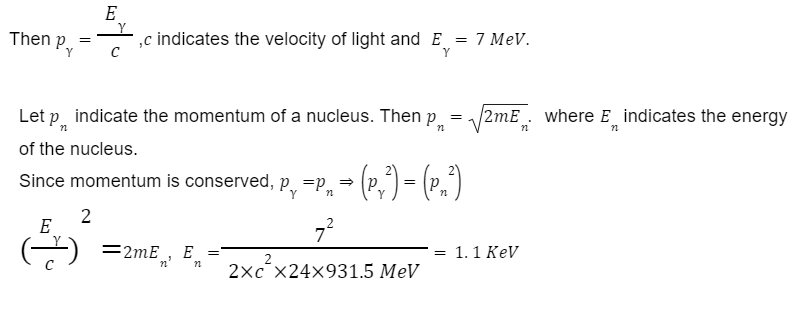Question.3 Calculate the frequency of a gamma ray photon that has a wavelength of 1.010-16 m ?

Answer)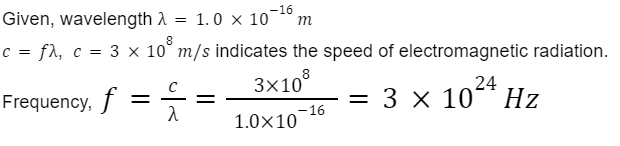Question.4 In a gamma decay reaction, the internal energy of a nucleus of mass M decreases, a gamma photon of energy E and linear momentum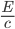is emitted and the nucleus recoils. Find the decrease in internal energy?

Mass of the photon=0, hence total momentum=0.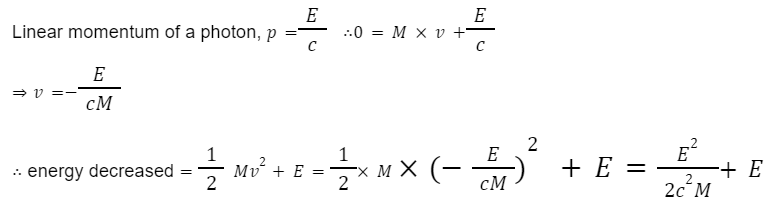## FAQs of Gamma Decay

Question.1 No change in atomic or mass number is observed when an element undergoes gamma decay. Why?

Answer. A change in atomic or mass number is observed when an element undergoes alpha (a) or (B) decay. During a gamma decay, only energetic photons are emitted.

Question.2 What particles are emitted during gamma decay?

Answer. Gamma photons having high energy are emitted during gamma decay.

Question.3 Gamma decay stabilizes the nucleus. Why?

Answer. The unstable nucleus returns to its ground state by dissipating some energy in the form of gamma photons, thus stabilizing the nucleus.

Question.4 Where are gamma rays used in the field of medicine?

Answer. Gamma rays are used to destroy cancer cells.

## NCERT Class 12 Physics Chapters

 Electric Charges And Fields Electrostatic Potential and Capacitance Current Electricity Moving Charges and Magnetism Magnetism and Matter Electromagnetic Induction Alternating Current Electromagnetic Waves Ray Optics and Optical Instruments Wave Optics Dual Nature of Radiation And Matter Atoms Nuclei Semiconductor Electronics Communication SystemsTalk to our expert
Resend OTP Timer =
By submitting up, I agree to receive all the Whatsapp communication on my registered number and Aakash terms and conditions and privacy policy